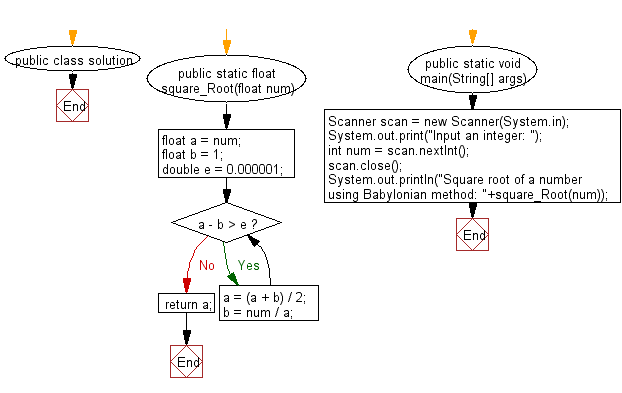﻿ Java Math Exercises: Find the square root of a number using Babylonian method - w3resource# Java Math Exercises: Find the square root of a number using Babylonian method

## Java Math Exercises: Exercise-14 with Solution

Write a Java program to find the square root of a number using Babylonian method.

Sample Solution:

Java Code:

``````import java.util.*;
public class solution {
public static float square_Root(float num)
{
float a = num;
float b = 1;
double e = 0.000001;
while (a - b > e) {
a = (a + b) / 2;
b = num / a;
}
return a;
}

public static void main(String[] args) {
Scanner scan = new Scanner(System.in);
System.out.print("Input an integer: ");
int num = scan.nextInt();
scan.close();
System.out.println("Square root of a number using Babylonian method: "+square_Root(num));
}
}
``````

Sample Output:

``` Input an integer:  25
Square root of a number using Babylonian method: 5.0
```

Flowchart:Java Code Editor:

What is the difficulty level of this exercise?

﻿

## Java: Tips of the Day

Parsing dates:

```import java.io.*;
import java.util.*;
import java.text.*;

String s = "2001/09/23 14:39";

SimpleDateFormat formatter = new SimpleDateFormat ("yyyy/MM/dd H:mm");
Date d = formatter.parse(s, new ParsePosition(0));
```